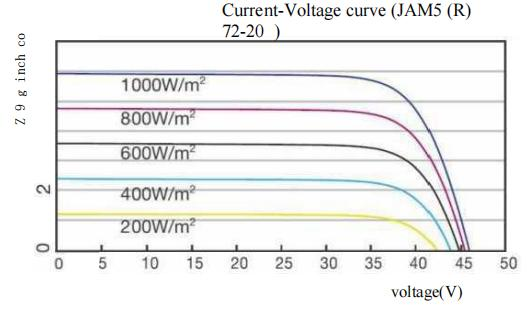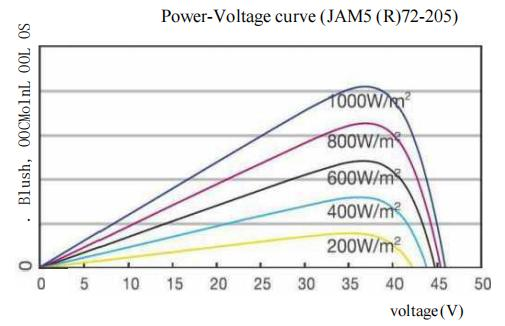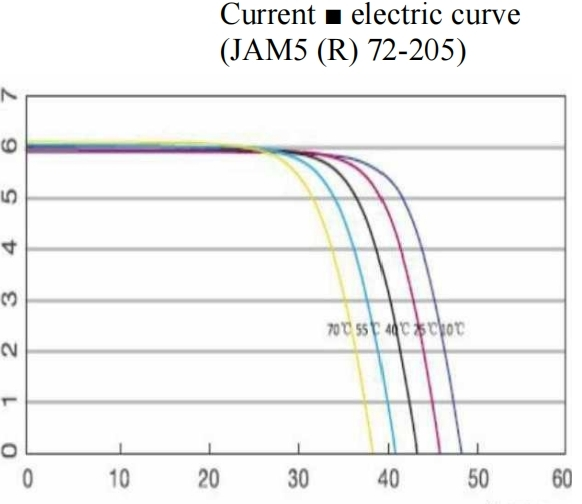﻿ Comparison of MPPT and PWM charging methods - Suzhou Cosuper Energy Technology Co., Ltd.
Search
×

# Comparison of MPPT and PWM charging methods

MPPT charging principle

MPPT (Maximum Power Point Tracking). Because the maximum output power point of the solar panel changes with the temperature, the MPPT function is required to detect the voltage of the solar panel port in real time, and track and control the optimal voltage of the solar panel port, so that the output voltage of the solar panel multiplied by the current reaches the maximum value ( That is, the output power of P=U*I reaches the maximum, so that the system charges the battery with the maximum power output. MPPT charging always keeps the port voltage of the solar panel at the best working voltage point of 36V, and always makes the solar panel output the maximum power, tracking Efficiency is larger than 99%.PWM charging principle

PWM charging is to force the solar panel port voltage down to the same as the battery port voltage.effectively, At certain ambient temperatures, the port voltage of the battery has gone far from the optimal operating voltage point of the solar panel, Power generation will be very inefficient,

See Figure 1, At an ambient temperature of 25 degrees, if the battery port voltage is 24V, Charging with a PWM, Equivalent to the solar panel output port voltage of 24V, As you can see from the figure above, Solar panels at 36V and 24V of the time, The output current is basically equal, So we can roughly calculate the tracking efficiency, Under the light of 1000W / M2 light exposure, The theoretical solar panels can output a power of: 36.5V * 6A=217W,

For PWM charging, the output power of the solar panel is: 24V * 6A=144W, tracking efficiency is: 144 / 217=66%.

Reasons why MPPT charging and PWM charging are comparable in efficiency in summerVoltage(V)

The temperature characteristics of solar panels is a very important

factor, as shown in the figure. When the temperature of the solar panels reaches 70 degrees (the summer temperature is basically 70 degrees), it is found that the best working voltage point is 27V. At this time, the theoretical solar panels can transmit the power is:

27V*6A=162W, PWM charging, the transmission power of solar panels is: 24V * 6A=144W, Tracking efficiency is: 144 / 162=89%.

It is not difficult to find that because of the high temperature in

summer, the best working voltage of solar panels drops to the tracking efficiency of PWM charging near the battery port voltage, which can be reached very high. At this time, the PWM charging efficiency can be comparable to that of MPPT charging efficiency.

The power of the final charge to the battery will also be related to the conversion efficiency of the controller. The conversion efficiency of PWM charging is very fixed. At 100% power, the conversion efficiency of MPPT is 96%. At 50% power, the conversion efficiency is 95%. At 25% power, the conversion efficiency is 93%. At 10% power, the conversion efficiency is 89%. At 5% power, the conversion efficiency is 84%.

Conclusion

• The PWM charging efficiency is greatly affected by the temperature of the solar panel. When the temperature is high (the temperature of the solar panel is basically above 60 degrees in summer), the operating voltage of the solar panel drops to a very low level, close to the battery port voltage, so It is not that the efficiency of MPPT charger is not high, but that when the PWM charger is charging at high temperature, the voltage of the battery is just close to the optimal operating voltage point of solar energy, and the charging efficiency is basically not much different from that of MPPT charging; but when the temperature is not high (such as winter), the charging efficiency is very low, and the charging efficiency is far behind the MPPT charging efficiency.

• In the cold winter, the light is not as good as in the summer, and high-efficiency charging is very necessary. The MPPT charger can maintain high-efficiency charging all the time, while the charging efficiency of the PWM charger is greatly reduced at this moment. In other words, when energy is most needed, the efficiency of PWM power generation is greatly reduced.

•  In areas where the temperature changes greatly throughout the year, MPPT charging is the best choice. In my country, MPPT charging is basically applicable. In terms of cost performance, PWM is more suitable for countries on the equator, such as Singapore, India and other temperatures. Somewhere high.

## RELATED COSUPER INSIGHTS & BLOG

• +86-0512-68638910

•